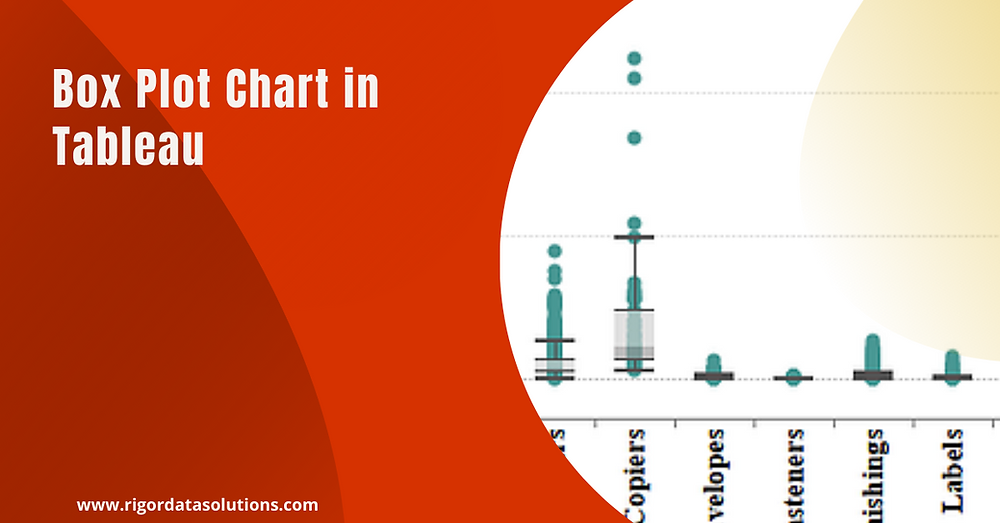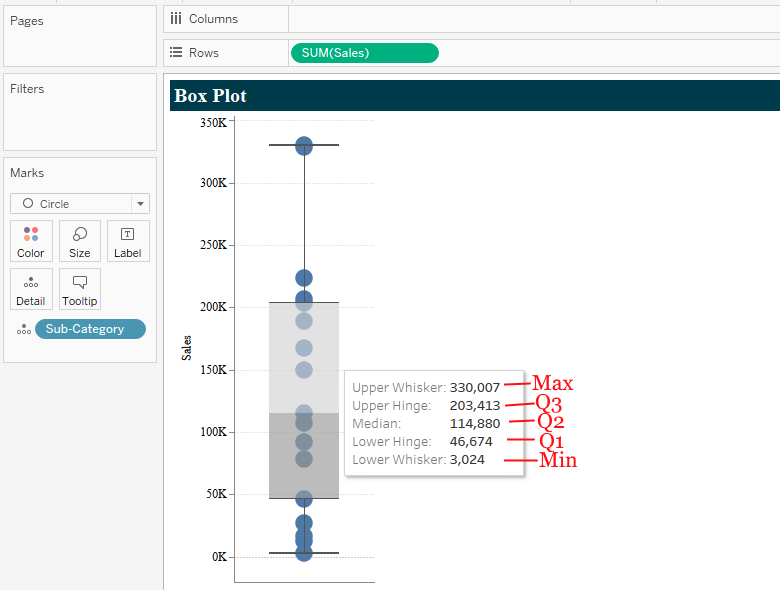Search
• rds team

# Tableau charts: Box plot

Updated: May 17, 2021### Definition

According to Wikipedia, box plot is a method of depicting groups of numerical data through their quartiles. Box plot may also have lines extending vertically from the boxes (whiskers) indicating the variability outside the upper and lower quartiles hence terms box and whisker plots. Outliers (Observation that is distant from other observations) may be plotted as individual points.

A sample of box plot diagram below.### Box plot best practices

• Always include axis for reference when interpreting the chart.

• Provide additional details like MAX, MIN, QUARTILE and MEDIAN values on the tooltip.

• Show the underlying marks - so to give the user perspective of where most of the data is concentrated. Use size of bubble to inform on the number of incidences a value occurs.

### Example of box plotUsing Superstores data set pre-packaged with Tableau app, we’ll seek to depict the distribution of Sales for Product Sub-Category.

### Step 1: Build a simple bar chart

• Drag dimension Sub-Category to the column shelf.

• Drag measure Sales to the row shelf. (Note, Sales is aggregated by SUM()).

Executing this, results to a simple bar chart.### Step 2: Make the box plot

• Under Show Me tab, choose box-and-whisker-plots as shown above.

Note, moving the cursor over the box displays a summary statistic as described in the box plot diagram at the beginning of this article.Looking carefully at the resulting chart, it shows distribution of Sales at Sub-Category summary level. Suppose, my interest is to see how Sales is distributed within the individual Sub-Category’s while still being able to compare distribution across other Sub-Category’s.

To effect this;

• Drag dimension Sub-Category to the row shelf.

• Under Analysis menu uncheck ‘Aggregate measures’.

See below.And there we’ve our box plot.

Using this box and whisker plot. Simple insights like distribution of Sales for different products Sub-Category’s can be spotted easily. For instance, using interquartile range, one can tell that the Sub-Category Machines and Copiers have the highest distribution of Sales. Outliers can also be spotted easily for different Sub-Category’s.